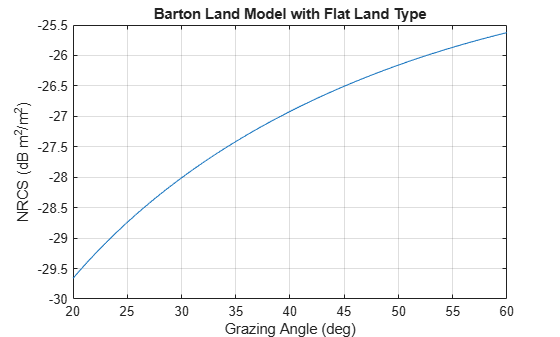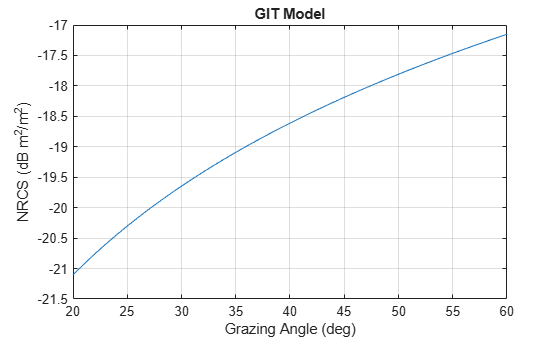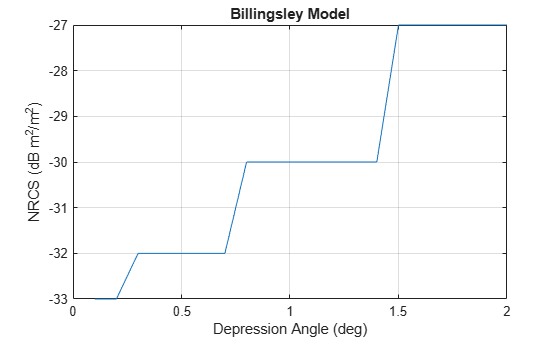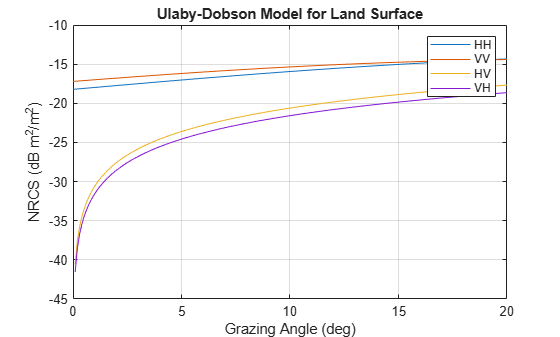# surfaceReflectivityLand

Normalized reflectivity of land surface

Since R2022a

## Description

This System object™ computes the normalized reflectivity of a land surface. Normalized reflectivity is the radar cross-section of a unit area of a surface. Multiplying by the total area of a surface or the illuminated area of a surface gives the total radar cross-section. Normalized reflectivity is also referred to as surface σ0 and is a function of frequency and grazing angle.

To compute the normalized reflectivity:

1. Create the `surfaceReflectivityLand` object and set its properties.

2. Call the object with arguments, as if it were a function.

## Creation

### Syntax

``refl = surfaceReflectivityLand``
``refl = surfaceReflectivityLand(Name=Value)``

### Description

example

````refl = surfaceReflectivityLand` creates a normalized reflectivity object `refl` for a land surface. Use this object to generate a normalized radar cross section (NRCS). This syntax creates a normalized reflectivity object with a `'Barton'` land `Model` and a `'Flatland'` `LandType`.It is not recommended to use models outside of their defined validity regions. See the `Model` property description for more information about available models and at what frequency and grazing angles the models are valid. See the `LandType` property help for information about applicable land types for each model.```

example

````refl = surfaceReflectivityLand(Name=Value)` creates a normalized reflectivity object for a land surface with the specified property `Name` set to the specified `Value`. You can specify additional name-value pair arguments in any order as (`Name1=Value1`, … ,`NameN=ValueN`).Example: ```refl = surfaceReflectivityLand(Model="GIT",LandType="Soil",SurfaceHeightStandardDeviation=1)``` creates a normalized reflectivity object for land using the GIT model with a `LandType` of `Soil` and a `SurfaceHeightStandardDeviation` of 1.```

## Properties

expand all

Unless otherwise indicated, properties are nontunable, which means you cannot change their values after calling the object. Objects lock when you call them, and the `release` function unlocks them.

If a property is tunable, you can change its value at any time.

Enable polarization, specified as `false` or `true`.

• When `true`, the System object enables the specification of cross-polarization components to help form a complete polarimetric normalized radar cross-section (NRCS) reflectivity matrix σ0 of the form

`${\sigma }^{0}=\left[\begin{array}{cc}{\sigma }_{HH}^{0}& {\sigma }_{HV}^{0}\\ {\sigma }_{VH}^{0}& {\sigma }_{VV}^{0}\end{array}\right]$`

where σ0HV and σ0VH are the cross-polarization components specified by the `ReflectivityHV` and `ReflectivityVH` properties, respectively. The remaining components, σ0HH and σ0VV, are determined by the particular model set by the `Model` property.

• When `false`, the System object lets you specify the mean polarization using the `Polarization` property based on the selected `Model`.

Cross-polarization components, specified as `'Full'` or `'Symmetric'`.

When the `CrossPolarization` property is set to `'Full'`:

• If the `Model` property is not set to `'ConstantGamma'`, the `ReflectivityHV` and `ReflectivityVH` properties are applicable, and unique speckle values are generated for all reflectivity components (σ0HH, σ0HV, σ0VH, and σ0VV).

• If the `Model` property set to `'ConstantGamma'`, the `CrossPolarization` property only affects speckle generation.

When the `CrossPolarization` property is set to `'Symmetric'` then reciprocity applies for monostatic geometry and the cross-polarization terms are equal, σ0HV = σ0VH.

• If the `Model` property is not `'ConstantGamma'`, only the `ReflectivityHV` property can be set. The `ReflectivityVH` property is assumed to be equal to the `ReflectivityHV` property. In addition, speckle values corresponding to the HV and VH components are equal.

• If the `Model` property is `'ConstantGamma'`, setting the `CrossPolarization` property to `'Symmetric'` requires that the HV and VH components of the `Gamma` property are equal and the object returns equal HV and VH speckle values.

#### Dependencies

To enable this property, set the `EnablePolarization` property to `true`.

Data Types: `char` | `string`

Land reflectivity model, specified as `'Barton'`, `'APL'`, `'Billingsley'`, `'GIT'`, `'Morchin'`, `'Nathanson'`, `'Nathanson'`, `'UlabyDobson'`, or `'ConstantGamma'`. Descriptions of the models and land types are shown in the table Land Reflectivity Models and Land Types.

Land type, specified as a char or string. The allowable land type and their default values depend on the `Model` property. If the `Model` property is not specified, the default land type is `'Flatland'`. Descriptions of the models and land types are shown in the table Land Reflectivity Models and Land Types.

Data Types: `char` | `string`

Standard deviation of the surface height, specified as a positive scalar. Units are in meters.

#### Dependencies

To enable this property, set the `SurfaceType` property to `'land'` and the `Model` property to `'GIT'`.

Data Types: `double`

Mean polarization of reflectivity model, specified as `'H'` or `'V'`. `'H'` designates horizontal polarization and `'V'` designates vertical polarization.

#### Dependencies

To enable this property, set the `Model` property to `'UlabyDobson'` and set the `EnablePolarization` property to `false`.

Data Types: `char` | `string`

Gamma value used in the constant gamma clutter reflectivity model, specified as a scalar or 2-by-2 real-valued matrix. The gamma value depends on both terrain type and operating frequency. The default value is representative of flat land. Units are in dB.

• If `EnablePolarization` is `false`, specify `Gamma` as a scalar.

• If `EnablePolarization` is `true`, specify `Gamma` as a scalar or a 2-by-2 matrix, such that Gamma = [GammaHH GammaHV; GammaVH GammaVV]. When specified as a scalar, it is assumed that all polarimetric components are equal.

To control the speckle values for the polarimetric `Gamma` case, set the `CrossPolarization` property to `'Full'` for unique speckle values for all components or `'Symmetric'` to set the speckle value for HV = VH.

Example: -15

#### Dependencies

To enable this property, set the `Model` property to `'ConstantGamma'`.

Data Types: `double`

Normalized radar cross section (NRCS), for the cross-polarized σ0HV component, where HV represents horizontal transmission and vertical reception. Q corresponds to the number of angles in either the `GrazingAngle` or `DepressionAngle` property. R corresponds to the number of frequencies in the `Frequency` property. The σ0HH and σ0VV components are derived from the selected model designated by the `Model` property and associated properties. Units are m²/m².

#### Dependencies

To enable this property, set the `EnablePolarization` property to `true` and set the `Model` property is anything other than `'ConstantGamma'`.

Data Types: `double`

Normalized radar cross section (NRCS), for the cross-polarized σ0VH component, where VH represents horizontal transmission and vertical reception. Q corresponds to the number of angles in either the `GrazingAngle` or `DepressionAngle` property. R corresponds to the number of frequencies in the `Frequency` property. The σ0HH and σ0VV components are derived from the selected model designated by the `Model` property and associated properties. Units are m²/m².

#### Dependencies

To enable this property, set the `CrossPolarization` property to `'Full'`.

Valid frequencies for the normalized reflectivity, specified as a length-R row vector. Frequency units are in Hz.

Example: `[1e6,10e6]`

#### Dependencies

To enable this property, set the `EnablePolarization` property to `true` and set the `Model` property to any value except `'Constant Gamma'`.

Data Types: `double`

Grazing angles, as a length-Q row vector. Units are in degrees.

Example: `[45:60]`

#### Dependencies

To enable this property, set the `EnablePolarization` property to `true` and set the `Model` property any value except `'Billingsley'` or `'Constant Gamma'`.

Data Types: `double`

Depression angles, specified as a length-Q row vector. Units are in degrees.

Example: [10:5:80]

#### Dependencies

To enable this property, set the `EnablePolarization` property to `true` and set the `Model` property to `'Billingsley'`.

Data Types: `double`

Speckle distribution type, specified as `'None'`, `'Lognormal'`, `'Rayleigh'`, or `'Weibull'`. Speckle is a multiplicative factor used to make clutter data appear noisier and is especially applicable to imaging applications.

Speckle is correlated with clutter RCS and is applied as I = σ*n, where σ represents the clutter RCS and n represents random numbers, which are often drawn from an independent identically-distributed unity mean noise statistical distribution.

• `None` – No speckle is applied.

• `Lognormal` – Speckle has a lognormal distribution. Define the distribution using the `SpeckleMean` and `SpeckleStandardDeviation` properties. Default values of these properties create speckle with a normalized mean lognormal distribution.

• `Rayleigh` – Speckle has a Rayleigh distribution. Define the distribution using the `SpeckleScale` property. The default value of this property creates speckle with a unit mean Rayleigh distribution.

• `Weibull` – Speckle has a Weibull distribution. Define the distribution using the `SpeckleScale` and `SpeckleShape` properties. The default values of these properties create speckle with a unit mean Rayleigh distribution.

Data Types: `char` | `string`

Mean value of lognormal-distributed speckle, specified as a scalar.

#### Dependencies

To enable this property, set the `Speckle` property to `'Lognormal'`.

Data Types: `double`

Standard deviation of lognormal-distributed speckle, specified as a non-negative scalar.

#### Dependencies

To enable this property, set the `Speckle` property to `'Lognormal'`.

Data Types: `double`

Scale parameter for speckle for the Rayleigh and Weibull distributions, specified as a positive scalar.

#### Dependencies

To enable this property, set the `Speckle` property to `'Rayleigh'` or `'Weibull'`.

Data Types: `double`

Shape value for the Weibull speckle distribution, specified as a positive scalar.

#### Dependencies

To enable this property, set the `Speckle` property to `'Weibull'`.

Data Types: `double`

## Usage

### Syntax

``nrcs = refl(ang,freq)``
``[nrcs,speck] = refl(graz,freq)``

### Description

example

````nrcs = refl(ang,freq)` returns the normalized radar cross section `nrcs` of a surface at angle `ang` and frequency `freq`. When the `Model` property is not set to `'Billingsley'`, `ang` is interpreted as a grazing angle. Then, it is interpreted as a depression angle.```

example

````[nrcs,speck] = refl(graz,freq)` also returns the multiplicative speckle `speck`.```

### Input Arguments

expand all

Grazing or depression angle of a surface relative to the radar, specified as a Q-length row vector of real values. When the land `Model` property is set to `'Billingsley'`, the angle is interpreted as a depression angle `depressionang` between –90° and 90°. For all other models, the angle is interpreted as a grazing angle `grazingang` ranging from 0° to 90°. Units are in degrees.

Transmitted frequencies, specified as a positive scalar or R-length vector of positive values. Units are in Hz.

Example: `freq = 70e9`

### Output Arguments

expand all

Normalized surface reflectivity , returned as a real-valued Q-by-R matrix or a real-valued 2-by-2-Q-by-R array. Q is the length of the grazing angle `graz` or, for land surfaces only, depression angle. R is the length of the frequency vector `freq`. `nrcs` is dimensionless but often expressed as m²/m². Normalized reflectivity is also called normalized radar cross section (NRCS).

• For the non-polarimetric reflectivity case, `nrcs` is returned as a real-valued Q-by-R matrix.

• For the polarimetric reflectivity case, `nrcs` is returned as a real-valued 2-b-2-byQ-by-R array. For each value of Q and R, `NRCS` forms a polarimetric normalized radar cross-section (NRCS) reflectivity matrix s of the form

`${\sigma }^{0}=\left[\begin{array}{cc}{\sigma }_{HH}^{0}& {\sigma }_{HV}^{0}\\ {\sigma }_{VH}^{0}& {\sigma }_{VV}^{0}\end{array}\right]$`

where SHV and SVH are the cross-polarization components specified by the `ReflectivityHV` and `ReflectivityVH` properties. The σ0HH and σ0VV components are derived from the specified model as set by the `Model` property

The returned normalized reflectivity for cross-polarization components σ0HV and σ0VH is calculated using nearest neighbor interpolation at a given grazing angle and frequency. To avoid interpolation errors, the normalized reflectivity values in the `ReflectivityHV` and `ReflectivityVH` properties should cover grazing angles from 0–90 degrees and all expected frequencies.

#### Dependencies

To enable the polarimetric reflectivity, set the `EnablePolarization` to `true`.

Multiplicative speckle, returned as a Q-by-R matrix where Q is the length of the angles vector in `ang` and R is the length of the frequency vector in `freq`. For the polarimetric reflectivity case, speckle is returned as a 2-by-2-by-Q-by-R MATLAB array.

Data Types: `double`

## Object Functions

To use an object function, specify the System object as the first input argument. For example, to release system resources of a System object named `obj`, use this syntax:

`release(obj)`

expand all

 `step` Run System object algorithm `release` Release resources and allow changes to System object property values and input characteristics `reset` Reset internal states of System object

## Examples

collapse all

Plot the normalized radar cross-section for grazing angles from 5 to 90 degrees. Assume the default 'Barton' land `Model` and 'Flatland' `LandType`. Set the radar frequency to 1 GHz.

```grazAng = 5:90; freq = 1e9; reflectivity = surfaceReflectivityLand; nrcs = reflectivity(grazAng,freq); plot(grazAng,pow2db(nrcs)) grid on xlabel('Grazing Angle (deg)') ylabel('NRCS (dB m^2/m^2)') title('Barton Land Model with Flat Land Type')```Configure a `radarscenario` to simulate a reflective land surface. Add a land surface object to define the physical properties of the scenario surface. The surface is a simple 200-by-200 meter rectangle. Use the `surfaceReflectivityLand` function to create a constant-gamma reflectivity model with a gamma value of -10 dB. Use the scenario `landSurface` method to add the rectangular land region and the radar reflectivity model to the scenario. Use a surface reference height of 16 meters.

```scene = radarScenario(UpdateRate = 0, IsEarthCentered = false); refl = surfaceReflectivityLand(Model = "ConstantGamma", Gamma = -10); srf = landSurface(scene,RadarReflectivity = refl, ... Boundary=[-100 100; -100 100],ReferenceHeight = 16)```
```srf = LandSurface with properties: RadarReflectivity: [1x1 surfaceReflectivityLand] ReflectivityMap: 1 ReferenceHeight: 16 Boundary: [2x2 double] Terrain: [] ```

Create a normalized reflectivity object using the GIT 'Model' and a 'Soil' land type. Obtain the normalized radar cross-section at a frequency of 3 GHz over grazing angles from 20 to 60 degrees. Assume a surface height standard deviation of two meters. Plot the surface reflectivity.

```grazAng = 20:60; freq = 10e9; reflectivity = surfaceReflectivityLand(Model="GIT", ... LandType="Soil",SurfaceHeightStandardDeviation=2); nrcs = reflectivity(grazAng,freq); plot(grazAng,pow2db(nrcs)) grid on xlabel('Grazing Angle (deg)') ylabel('NRCS (dB m^2/m^2)') title('GIT Model')```Create a normalized reflectivity object using the Billingsley 'Model' and a 'LowReliefRural' land type. Obtain the normalized radar cross-section at a frequency of 3 GHz over depression angles from 0.1 to 3 degrees. Plot the surface reflectivity.

``` depAng = 0.1:0.1:2; freq = 3e9; reflectivity = surfaceReflectivityLand(Model="Billingsley", ... LandType="LowReliefRural"); nrcs = reflectivity(depAng,freq); plot(depAng,pow2db(nrcs)) grid on xlabel('Depression Angle (deg)') ylabel('NRCS (dB m^2/m^2)') title('Billingsley Model')```Create a normalized reflectivity object using the Ulaby-Dobson model for a grass land type. Obtain the normalized radar cross-section for both vertical and horizontal polarizations at a frequency of 10 GHz over grazing angles from 1 to 10 degrees. Plot the surface reflectivities.

```grazAng = 1:0.1:10; freq = 10e9; reflectivity_v = surfaceReflectivityLand(Model="UlabyDobson", ... LandType="Grass",Polarization="V"); nrcs_v = reflectivity_v(grazAng,freq); reflectivity_h = surfaceReflectivityLand(Model="UlabyDobson", ... LandType="Grass",Polarization="H"); nrcs_h = reflectivity_h(grazAng,freq); plot(grazAng,pow2db(nrcs_v)) hold on plot(grazAng,pow2db(nrcs_h)) grid on legend('Vertical Polarization','Horizonal Polarization') xlabel('Grazing Angle (deg)') ylabel('NRCS (dB m^2/m^2)') title('Ulaby-Dobson Model')```Create a surface with two hills. Plot the surface on a 200-by-200 meter grid with grid points one meter apart. Add the surface to a radar scenario. Assume the surface has a radar reflectivity defined by a constant gamma model.

```[x,y] = meshgrid(linspace(-100,100,201)); ht1 = 40*exp(-(x.^2 + y.^2)/30^2); ht2 = 100*exp(-((x-60).^2 + y.^2)/25^2); ht = ht1 + ht2; p = surfc(x(1,:),y(:,1),ht); axis equal axis tight shading interp simTime = 3; scene = radarScenario(UpdateRate = 1, ... IsEarthCentered = false,StopTime = simTime); gammaDB = surfacegamma('Flatland'); refl = surfaceReflectivityLand(Model = 'ConstantGamma',Gamma = gammaDB); srf = landSurface(scene,RadarReflectivity = refl, ... Terrain = ht,Boundary = [-100,100;-100,100]);```

Use surface manager to identify the surface.

`scene.SurfaceManager`
```ans = SurfaceManager with properties: UseOcclusion: 1 Surfaces: [1x1 radar.scenario.LandSurface] ```
`scene.SurfaceManager.Surfaces`
```ans = LandSurface with properties: RadarReflectivity: [1x1 surfaceReflectivityLand] ReflectivityMap: 1 ReferenceHeight: 0 Boundary: [2x2 double] Terrain: [201x201 double] ```

Obtain and plot the height of the surface at the point (50,-30).

```xt = 50; yt = -30; htx = height(srf,[xt,yt])```
```htx = 21.1046 ```
```hold on plot3(xt,yt,htx+5,'ow','MarkerFaceColor','r') xlabel('x') ylabel('y') hold off```Create a land normalized reflectivity object using the Ulaby-Dobson model and a grass land type. Enable polarization and specify the cross-polarization reflectivity. Obtain the NRCS at a frequency of 10 GHz over grazing angles from 1 to 10 degrees. Plot the reflectivities.

```grazAng = 0:0.1:20; freq = 10e9; surf = surfaceReflectivityLand(Model='UlabyDobson', ... LandType='Grass',EnablePolarization=true, ... GrazingAngle=0:.1:90,Frequency=[100,1e6,11e6], ... ReflectivityHV=0.05*sind(0:.1:90)'*[1 1 1], ... ReflectivityVH=0.04*sind(0:.1:90)'*[1 1 1])```
```surf = surfaceReflectivityLand with properties: EnablePolarization: 1 CrossPolarization: 'Full' Model: 'UlabyDobson' LandType: 'Grass' ReflectivityHV: [901x3 double] ReflectivityVH: [901x3 double] Frequency: [100 1000000 11000000] GrazingAngle: [0 0.1000 0.2000 0.3000 0.4000 0.5000 0.6000 0.7000 0.8000 0.9000 1 1.1000 1.2000 1.3000 1.4000 1.5000 1.6000 1.7000 1.8000 1.9000 2 2.1000 2.2000 2.3000 2.4000 2.5000 2.6000 2.7000 2.8000 2.9000 3 3.1000 3.2000 3.3000 ... ] Speckle: 'None' ```
```nrcs = surf(grazAng,freq); plot(grazAng,pow2db(squeeze(nrcs(1,1,:))), ... grazAng,pow2db(squeeze(nrcs(2,2,:))), ... grazAng,pow2db(squeeze(nrcs(1,2,:))), ... grazAng,pow2db(squeeze(nrcs(2,1,:)))) legend('HH','VV','HV','VH'); grid on xlabel('Grazing Angle (deg)') ylabel('NRCS (dB m^2/m^2)') title('Ulaby-Dobson Model for Land Surface')```expand all

 Barton, David Knox. Radar Equations for Modern Radar. Artech House, 2013.

 Long, Maurice W. Radar Reflectivity of Land and Sea. 3rd ed, Artech House, 2001.

 Nathanson, Fred E., et al. Radar Design Principles: Signal Processing and the Environment. 2. ed., Repr, Scitech Publ, 2004.

 Reilly, J. P., R. L. McDonald, and G. D. Dockery. "RF-Environment Models for the ADSAM Program." Report No. A1A97U-070, Laurel, MD: Johns Hopkins University Applied Physics Laboratory, August 22, 1997.

 Billingsley, J. Barrie. Low-Angle Radar Land Clutter: Measurements and Empirical Models. William Andrew Pub. : SciTech Pub. ; Institution of Electrical Engineers, 2002.

 Richards, M. A., et al., editors. Principles of Modern Radar. SciTech Pub, 2010.

 Morchin, Fred E., J. Patrick Reilly, and Marvin Cohen. Radar Design Principles: Signal Processing and the Environment. 2nd ed. New York: McGraw-Hill, 1991.

 Ulaby, Fawwaz T., and M. Craig Dobson. Handbook of Radar Scattering Statistics for Terrain. Artech House, 1989.